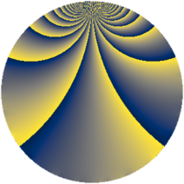# Properties

 Label 1210.2.jLevel $1210$ Weight $2$ Character orbit 1210.j Rep. character $\chi_{1210}(9,\cdot)$ Character field $\Q(\zeta_{10})$ Dimension $216$ Sturm bound $396$

# Related objects

## Defining parameters

 Level: $$N$$ $$=$$ $$1210 = 2 \cdot 5 \cdot 11^{2}$$ Weight: $$k$$ $$=$$ $$2$$ Character orbit: $$[\chi]$$ $$=$$ 1210.j (of order $$10$$ and degree $$4$$) Character conductor: $$\operatorname{cond}(\chi)$$ $$=$$ $$55$$ Character field: $$\Q(\zeta_{10})$$ Sturm bound: $$396$$

## Dimensions

The following table gives the dimensions of various subspaces of $$M_{2}(1210, [\chi])$$.

Total New Old
Modular forms 888 216 672
Cusp forms 696 216 480
Eisenstein series 192 0 192

## Trace form

 $$216q + 54q^{4} - 4q^{6} + 74q^{9} + O(q^{10})$$ $$216q + 54q^{4} - 4q^{6} + 74q^{9} + 4q^{10} + 6q^{14} - 14q^{15} - 54q^{16} + 16q^{19} + 40q^{21} + 4q^{24} + 12q^{25} + 4q^{26} + 4q^{29} + 26q^{30} - 4q^{31} - 8q^{34} + 52q^{35} - 54q^{36} + 36q^{39} + 6q^{40} - 46q^{41} - 52q^{45} - 32q^{46} - 8q^{49} + 4q^{50} - 12q^{51} - 64q^{54} + 4q^{56} + 4q^{59} - 16q^{60} - 56q^{61} + 54q^{64} - 44q^{65} - 48q^{69} - 16q^{70} + 80q^{71} - 56q^{75} + 4q^{76} + 4q^{79} - 10q^{80} + 86q^{81} + 20q^{84} + 38q^{85} + 8q^{86} + 188q^{89} - 10q^{90} - 2q^{91} + 30q^{94} + 50q^{95} - 4q^{96} + O(q^{100})$$

## Decomposition of $$S_{2}^{\mathrm{new}}(1210, [\chi])$$ into newform subspaces

The newforms in this space have not yet been added to the LMFDB.

## Decomposition of $$S_{2}^{\mathrm{old}}(1210, [\chi])$$ into lower level spaces

$$S_{2}^{\mathrm{old}}(1210, [\chi]) \cong$$ $$S_{2}^{\mathrm{new}}(55, [\chi])$$$$^{\oplus 4}$$$$\oplus$$$$S_{2}^{\mathrm{new}}(110, [\chi])$$$$^{\oplus 2}$$$$\oplus$$$$S_{2}^{\mathrm{new}}(605, [\chi])$$$$^{\oplus 2}$$